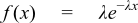#Boost C++ Libraries

...one of the most highly regarded and expertly designed C++ library projects in the world.

This is the documentation for an old version of boost. Click here for the latest Boost documentation.
##### Exponential Distribution

`#include <boost/math/distributions/exponential.hpp>`

```template <class RealType = double,
class Policy   = policies::policy<> >
class exponential_distribution;

typedef exponential_distribution<> exponential;

template <class RealType, class Policy>
class exponential_distribution
{
public:
typedef RealType value_type;
typedef Policy   policy_type;

exponential_distribution(RealType lambda = 1);

RealType lambda()const;
};
```It is often used to model the time between independent events that happen at a constant average rate.

The following graph shows how the distribution changes for different values of the rate parameter lambda:##### Member Functions
```exponential_distribution(RealType lambda = 1);
```

Constructs an Exponential distribution with parameter lambda. Lambda is defined as the reciprocal of the scale parameter.

Requires lambda > 0, otherwise calls domain_error.

```RealType lambda()const;
```

Accessor function returns the lambda parameter of the distribution.

##### Non-member Accessors

All the usual non-member accessor functions that are generic to all distributions are supported: Cumulative Distribution Function, Probability Density Function, Quantile, Hazard Function, Cumulative Hazard Function, mean, median, mode, variance, standard deviation, skewness, kurtosis, kurtosis_excess, range and support.

The domain of the random variable is [0, +∞].

##### Accuracy

The exponential distribution is implemented in terms of the standard library functions `exp`, `log`, `log1p` and `expm1` and as such should have very low error rates.

##### Implementation

In the following table λ is the parameter lambda of the distribution, x is the random variate, p is the probability and q = 1-p.

Function

Implementation Notes

pdf

Using the relation: pdf = λ * exp(-λ * x)

cdf

Using the relation: p = 1 - exp(-x * λ) = -expm1(-x * λ)

cdf complement

Using the relation: q = exp(-x * λ)

quantile

Using the relation: x = -log(1-p) / λ = -log1p(-p) / λ

quantile from the complement

Using the relation: x = -log(q) / λ

mean

1/λ

standard deviation

1/λ

mode

0

skewness

2

kurtosis

9

kurtosis excess

6

##### references

(See also the reference documentation for the related Extreme Distributions.)

 Copyright © 2006 , 2007, 2008, 2009, 2010 John Maddock, Paul A. Bristow, Hubert Holin, Xiaogang Zhang, Bruno Lalande, Johan Råde, Gautam Sewani and Thijs van den Berg Distributed under the Boost Software License, Version 1.0. (See accompanying file LICENSE_1_0.txt or copy at http://www.boost.org/LICENSE_1_0.txt)